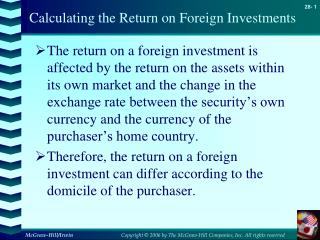# Calculating the Return on Foreign Investments - PowerPoint PPT PresentationDownload PresentationCalculating the Return on Foreign Investments

Calculating the Return on Foreign InvestmentsDownload Presentation## Calculating the Return on Foreign Investments

- - - - - - - - - - - - - - - - - - - - - - - - - - - E N D - - - - - - - - - - - - - - - - - - - - - - - - - - -
##### Presentation Transcript

1. Calculating the Return on Foreign Investments • The return on a foreign investment is affected by the return on the assets within its own market and the change in the exchange rate between the security’s own currency and the currency of the purchaser’s home country. • Therefore, the return on a foreign investment can differ according to the domicile of the purchaser.

2. Calculating the Return on Foreign Investments • From the viewpoint of an American investor, assume the following information :

3. Calculating the Return on Foreign Investments • The return to a Mexican investor expressed in his home currency (pesos) is : • The return to a US investor on Mexican stocks is :

4. Calculating the Return on Foreign Investments • Dividing the return to the American investor into a component due to return in the Mexican market and the return due to exchange gains or losses gives : where Rx is the exchange return C’est-à-dire la variation en % du taux de change

5. 0 • By approximation, we have the following expressions for expected return and standard deviation of return on a foreign security : Calculating the Return and Risk on Foreign Investments • Restating the preceding equation : • Simplifying : NB : la formule qui permet de calculer ici le risque d’un actif financier étranger est identique à celle qui nous a permis de calculer le risque d’un portefeuille composé de deux actifs (cf. Chapitre 2, slides 25 - 28)

6. Calculating the Return and Risk on Foreign Investments • Usually, there is a very low correlation between exchange returns (gains or losses expressed as %) and market returns in a country. Therefore, the covariance between exchange returns and market returns is close to zero, so :

7. Thus, the standard deviation of the return expressed in dollars is considerably less than the sum of the standard deviation of the exchange return and the standard deviation of the return of the security in its home currency (here, in pesos) Calculating the Return and Risk on Foreign Investments • Let : • Then :

8. The Rationale of Holding Foreign Securities in a Portfolio • Holding foreign (Mexican) securities -from the US standpoint- as long as : =bMEX Where : Expected return on Mexican securities in dollars Expected return on US securities Standard deviation of the Mexican securities in dollars Standard deviation of US securities Coefficient of correlation between US securities returns and Mexican securities US Risk free rate of interest